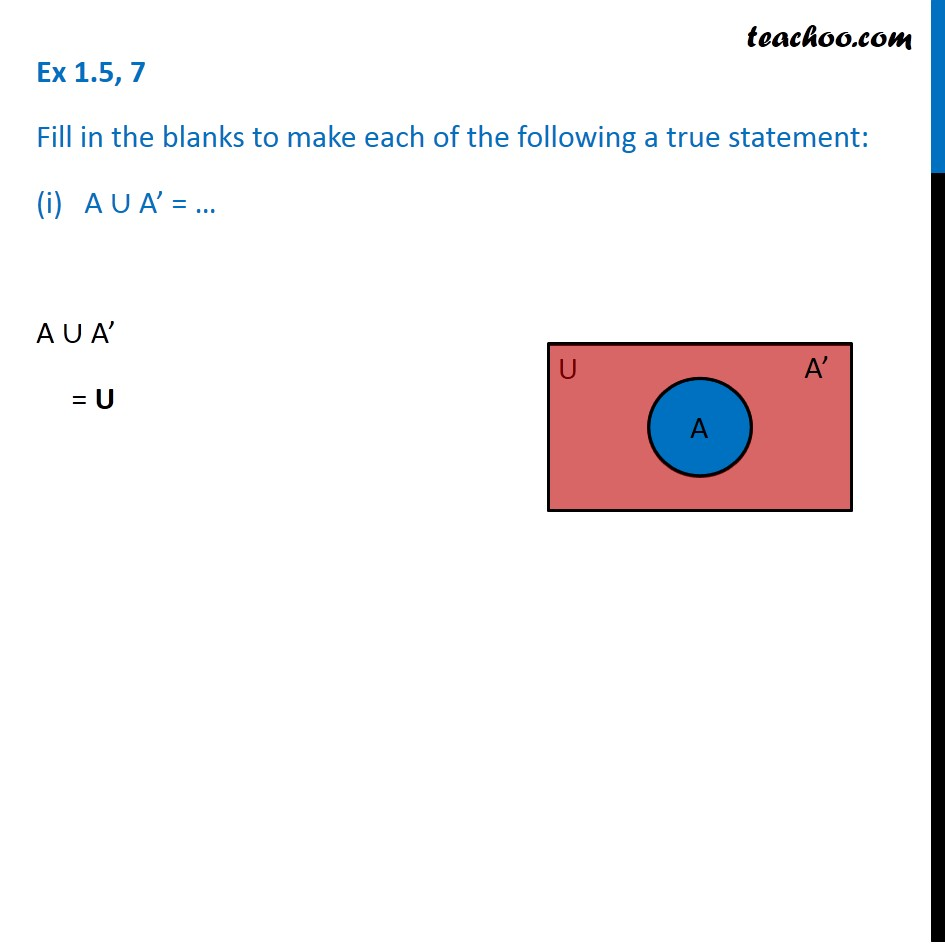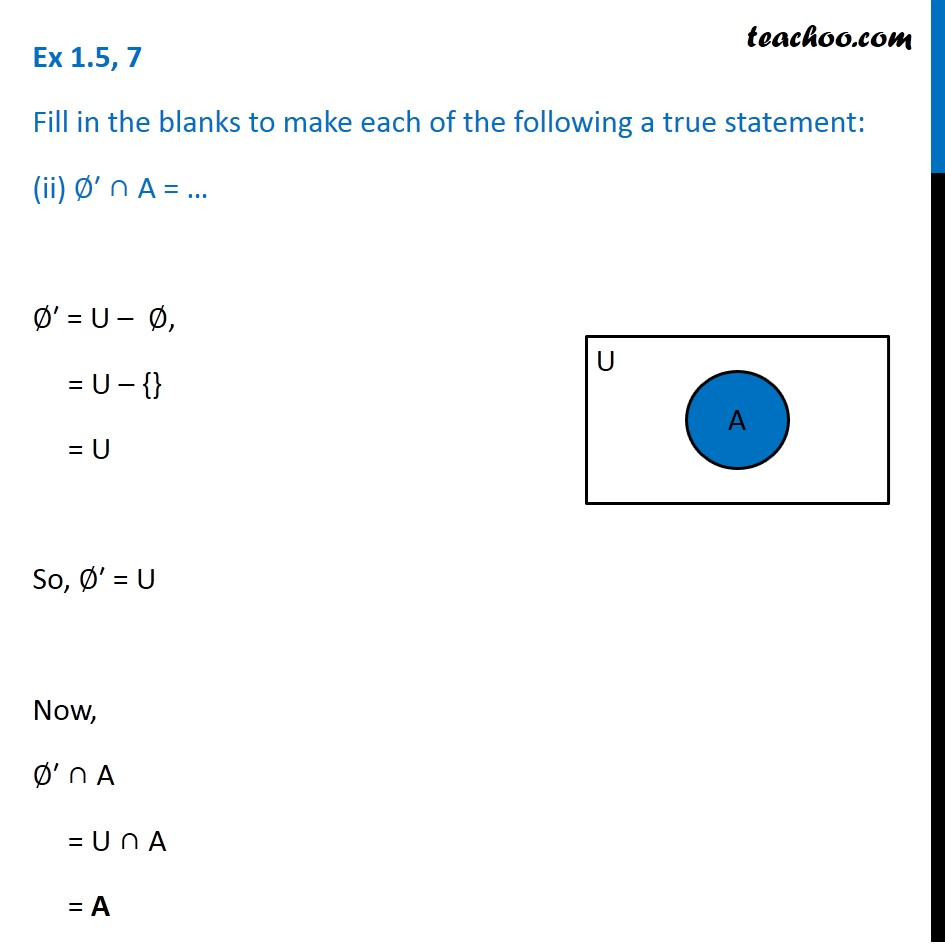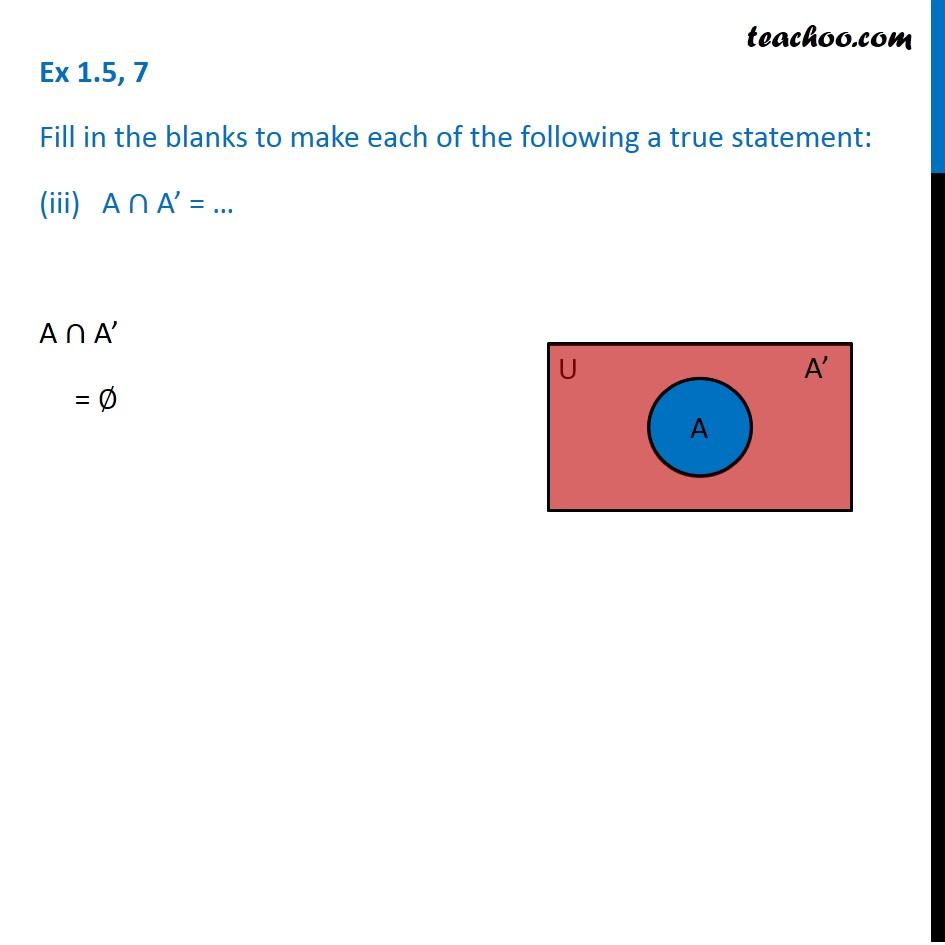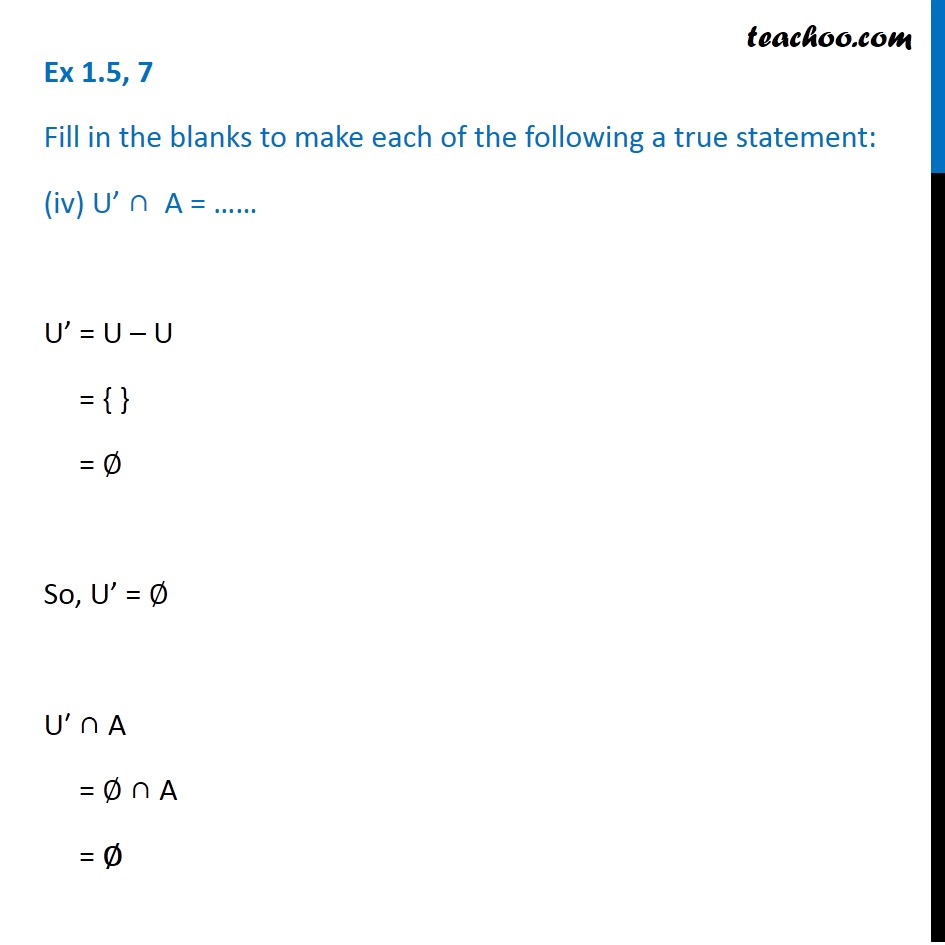1. Chapter 1 Class 11 Sets
2. Concept wise
3. Complement of set

Transcript

Ex 1.5, 7 Fill in the blanks to make each of the following a true statement: (i) A ∪ A’ = … A ∪ A’ = U Ex 1.5, 7 Fill in the blanks to make each of the following a true statement: (ii) ∅′ ∩ A = … ∅′ = U – ∅, = U – {} = U So, ∅′ = U Now, ∅′ ∩ A = U ∩ A = A Ex 1.5, 7 Fill in the blanks to make each of the following a true statement: (iii) A ∩ A’ = … A ∩ A’ = ∅ Ex 1.5, 7 Fill in the blanks to make each of the following a true statement: (iv) U’ ∩ A = …… U’ = U – U = { } = ∅ So, U’ = ∅ U′ ∩ A = ∅ ∩ A = ∅

Complement of set